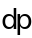#Don Park

### Site Tools

orbitalmechanics

1 Newton = 1 kg * m/s^2

== Vehicle

80kg (175 lbs)

80kg in earth's gravity (9.8m/s^2) creates 784 Newtons of downward force.

== Motor

1,000 N engine (enough to overcome rocket weight)

== Launch

The upward force is 216N.

The motor runs for 1 second.

== Questions

What is the speed of the vehicle?

216N * 1s = 216 kg m/s (Unit of momentum)

216kg m/s / 80 kg = 2.7m/s

What is the altitude of the vehicle?

position = acceleration * time^2 / 2 (from two rounds of integration)

2.7 * 1^2 / 2 = 1.45m